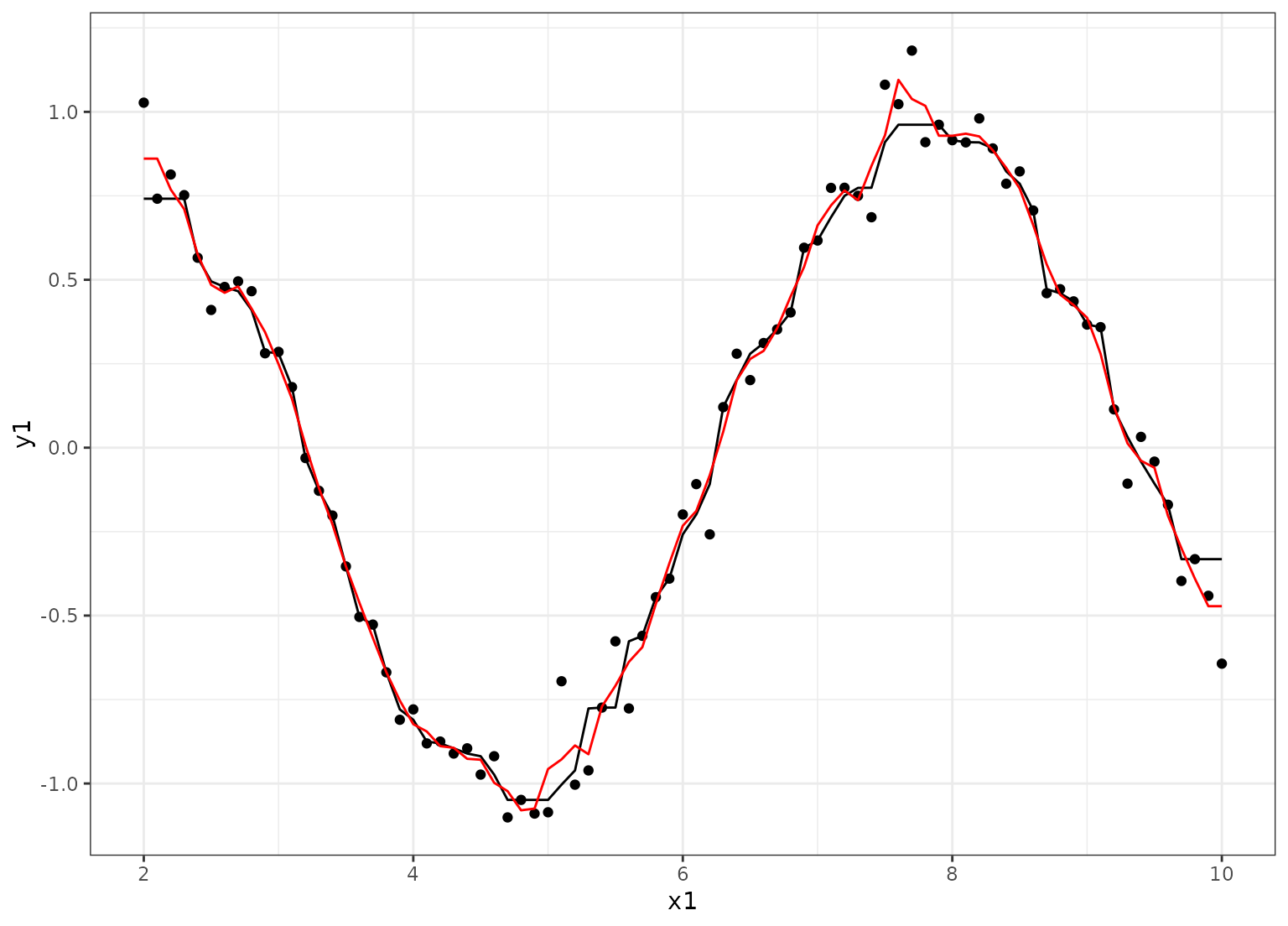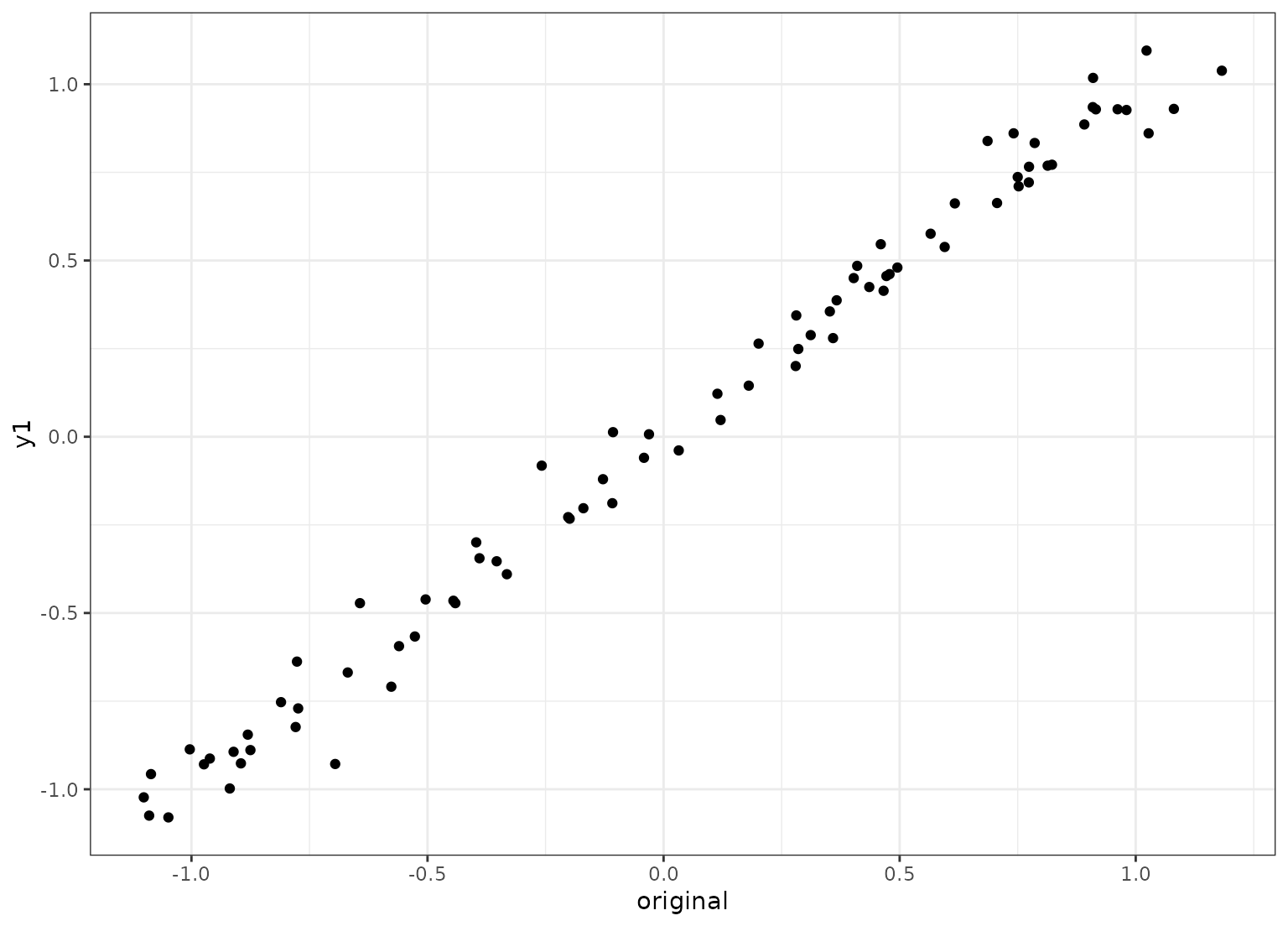step_window creates a specification of a recipe step that will create new columns that are the results of functions that compute statistics across moving windows.

step_window(
recipe,
...,
role = NA,
trained = FALSE,
size = 3,
na_rm = TRUE,
statistic = "mean",
columns = NULL,
names = NULL,
skip = FALSE,
id = rand_id("window")
)

## Arguments

recipe A recipe object. The step will be added to the sequence of operations for this recipe. One or more selector functions to choose variables for this step. See selections() for more details. For model terms created by this step, what analysis role should they be assigned? If names is left to be NULL, the rolling statistics replace the original columns and the roles are left unchanged. If names is set, those new columns will have a role of NULL unless this argument has a value. A logical to indicate if the quantities for preprocessing have been estimated. An odd integer >= 3 for the window size. A logical for whether missing values should be removed from the calculations within each window. A character string for the type of statistic that should be calculated for each moving window. Possible values are: 'max', 'mean', 'median', 'min', 'prod', 'sd', 'sum', 'var' A character string that contains the names of columns that should be processed. These values are not determined until prep.recipe() is called. An optional character string that is the same length of the number of terms selected by terms. If you are not sure what columns will be selected, use the summary function (see the example below). These will be the names of the new columns created by the step. A logical. Should the step be skipped when the recipe is baked by bake.recipe()? While all operations are baked when prep.recipe() is run, some operations may not be able to be conducted on new data (e.g. processing the outcome variable(s)). Care should be taken when using skip = TRUE as it may affect the computations for subsequent operations. A character string that is unique to this step to identify it.

## Value

An updated version of recipe with the new step added to the sequence of any existing operations.

## Details

The calculations use a somewhat atypical method for handling the beginning and end parts of the rolling statistics. The process starts with the center justified window calculations and the beginning and ending parts of the rolling values are determined using the first and last rolling values, respectively. For example, if a column x with 12 values is smoothed with a 5-point moving median, the first three smoothed values are estimated by median(x[1:5]) and the fourth uses median(x[2:6]).

step will stop with a note about installing the package.

When you tidy() this step, a tibble with columns terms (the selectors or variables selected), statistic (the summary function name), and size is returned.

## Examples

library(recipes)
library(dplyr)
library(rlang)
library(ggplot2, quietly = TRUE)

set.seed(5522)
sim_dat <- data.frame(x1 = (20:100) / 10)
n <- nrow(sim_dat)
sim_dat$y1 <- sin(sim_dat$x1) + rnorm(n, sd = 0.1)
sim_dat$y2 <- cos(sim_dat$x1) + rnorm(n, sd = 0.1)
sim_dat$x2 <- runif(n) sim_dat$x3 <- rnorm(n)

rec <- recipe(y1 + y2 ~ x1 + x2 + x3, data = sim_dat) %>%
step_window(starts_with("y"), size = 7, statistic = "median",
names = paste0("med_7pt_", 1:2),
role = "outcome") %>%
step_window(starts_with("y"),
names = paste0("mean_3pt_", 1:2),
role = "outcome")
rec <- prep(rec, training = sim_dat)

smoothed_dat <- bake(rec, sim_dat, everything())

ggplot(data = sim_dat, aes(x = x1, y = y1)) +
geom_point() +
geom_line(data = smoothed_dat, aes(y = med_7pt_1)) +
geom_line(data = smoothed_dat, aes(y = mean_3pt_1), col = "red") +
theme_bw()tidy(rec, number = 1)
#> # A tibble: 2 × 4
#>   terms statistic  size id
#>   <chr> <chr>     <int> <chr>
#> 1 y1    median        7 window_MbmJQ
#> 2 y2    median        7 window_MbmJQ
tidy(rec, number = 2)
#> # A tibble: 2 × 4
#>   terms statistic  size id
#>   <chr> <chr>     <int> <chr>
#> 1 y1    mean          3 window_uDjJs
#> 2 y2    mean          3 window_uDjJs

# If you want to replace the selected variables with the rolling statistic
# don't set names
sim_dat$original <- sim_dat$y1
rec <- recipe(y1 + y2 + original ~ x1 + x2 + x3, data = sim_dat) %>%
step_window(starts_with("y"))
rec <- prep(rec, training = sim_dat)
smoothed_dat <- bake(rec, sim_dat, everything())
ggplot(smoothed_dat, aes(x = original, y = y1)) +
geom_point() +
theme_bw()